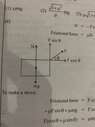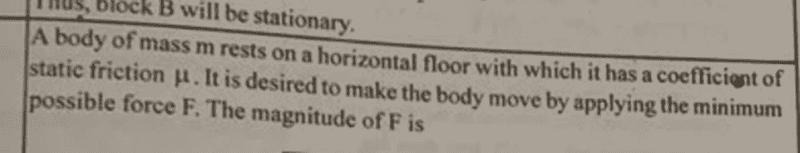# Minimum force to move a block

• rudransh verma

#### rudransh verma

Gold Member
Homework Statement
A body of mass m results on horizontal floor with which it has coefficient of static friction ##\mu##. It is desired to move the block with minimum possible force. Find the applied force F.
Relevant Equations
##F_n=ma##
##F_s=\mu F_N##
We know the minimum force to move the body in presence of friction will be ##F>\mu F_N##
But it’s not right.

We know the minimum force to move the body in presence of friction will be ##F>\mu F_N##
But it’s not right.
Why not? (What does ##F_N## equal?)

Where is the effort here?

•Tesla In Person
I am intrigued, what is the answer key @rudransh verma . Why you say its not correct? According to my opinion the minimum force is ##\mu mg##.

•jbriggs444
Why not? (What does ##F_N## equal?)
I am intrigued, what is the answer key @rudransh verma . Why you say its not correct? According to my opinion the minimum force is ##\mu mg##.
Yes ##\mu mg##. But the answer in my book says ##F=\frac{\mu mg}{\sqrt{1+\mu^2}}##

Yes ##\mu mg##. But the answer in my book says ##F=\frac{\mu mg}{\sqrt{1+\mu^2}}##
Something is fishy here, can you post some sort of figure that perhaps exists in the book for this problem?

But the answer in my book says ##F=\frac{\mu mg}{\sqrt{1+\mu^2}}##
Did you provide the complete problem statement?

•rudransh verma
Something is fishy here, can you post some sort of figure that perhaps exists in the book for this problem?

#### Attachments

•image.jpg
25.2 KB · Views: 70
Seriously? You only now thought it relevant to tell us that the applied force F is at an angle?

••Steve4Physics, Delta2, berkeman and 1 other person
Seriously? You only now thought it relevant to tell us that the applied force F is at an angle?
No. This is the complete question. There is no mentioning of any angle as you can see.I don’t know why they are applying force at an angle.

No. This is the complete question. There is no mentioning of any angle as you can see.I don’t know why they are applying force at an angle.
So you are saying that the diagram you just posted has nothing to do with this problem?

•So you are saying that the diagram you just posted has nothing to do with this problem?
I am saying they have solved the problem assuming force at an angle. That diagram is not the part of question but of the answer.

I am saying they have solved the problem assuming force at an angle. That diagram is not the part of question but of the answer.
That's a bit hard to believe.

(1) Post the complete problem statement
(2) Post the complete solution provided.

••Ah, I see the issue now. You were not told anything about the direction of the applied force, so you are free to apply it in any direction to solve for the minimum force needed. Assuming that the applied force must be horizontal was a mistake.

•vcsharp2003
See my last post. Do you see how being allowed to apply the force at an angle can affect the answer?

I don’t know why they are applying force at an angle.
Because that lowers the normal force and therefore the horizontal force required to move the block. Since the problem did not say anything about the direction, in order to find the minimal force, you need to find an expression for the required force as a function of the angle and then minimise with respect to the angle.

••Delta2
OK , well I am not sure if that "the force applied must be at an angle " should be part of the problem statement or the student would have to think it. I was not smart enough to think it, and I am deeply sorry for myself.

••vcsharp2003 and sysprog
OK , well I am not sure if that "the force applied must be at an angle " should be part of the problem statement or the student would have to think it. I was not smart enough to think it, and I am deeply sorry for myself.
Everybody makes bloopers or misread problems sometimes. Obviously, stating explicitly that the force could be at an angle would make it more obvious, but technically it is also fair game with the problem just stating to find the minimal force.

•Delta2, vcsharp2003, Doc Al and 1 other person
Obviously, stating explicitly that the force could be at an angle would make it more obvious, but technically it is also fair game with the problem just stating to find the minimal force.
The problem asks specifically for the magnitude of the force. That's a very broad hint here.

•vcsharp2003 and vela
OK , well I am not sure if that "the force applied must be at an angle " should be part of the problem statement or the student would have to think it. I was not smart enough to think it, and I am deeply sorry for myself.
It can help to put yourself into the problem. If you had to tug a heavy mass across a floor, how would you do it?

•Nugatory
It can help to put yourself into the problem. If you had to tug a heavy mass across a floor, how would you do it?
I don't know how you think but according to my thinking and intuition I would push it with horizontal force.

•Tom.G and russ_watters
I don't know how you think but according to my thinking and intuition I would push it with horizontal force.
So you just learned something practically useful!In addition, pulling at an angle will also mean helping to overcome issues of bad grip on the ground on your part as you also increase your own normal force.

•russ_watters and jbriggs444
I don't know how you think but according to my thinking and intuition I would push it with horizontal force.
Maybe you need to do more manual labour!

Two physicists were watching workmen on a building site. "See how he pulls the wheelbarrow behind himself instead of pushing it in front. He has obviously worked out that because he is pulling up at a slight angle it reduces the rolling resistance", observed one.
The other physicist asked the workman why he did it. "I've pushed wheelbarrows for thirty years," he replied, "and I'm fed up with the sight of 'em."

Last edited:
••phinds, Nugatory, russ_watters and 6 others
Because that lowers the normal force and therefore the horizontal force required to move the block.
To apply minimum force the force of friction has to be less and will become less when we decrease the normal force and that would mean a tilted block.
But the eqns are ##F_N+F\sin \theta =mg##
##F\cos \theta=\mu F_N## which I don’t think is consistent with the tilted block.

and that would mean a tilted block.
No, it would not necessarily tilt the block. Why do you think so? There is a separate set of equations (based on torque balance) that would tell you whether the block tilts or not.

which I don’t think is consistent with the tilted block.
Why not? They are valid as long as the coefficient of friction remains the same.

No, it would not necessarily tilt the block. Why do you think so?
If it will not leave the ground at least from one side how the friction will decrease ?

If it will not leave the ground at least from one side how the friction will decrease ?
By decreasing the normal force. Maximal friction is proportional to normal force.

•russ_watters
By decreasing the normal force. Maximal friction is proportional to normal force.
Are you saying applying a force at an angle can decrease the friction without actually lifting it. That there is some ##0<F_N<mg##

Are you saying applying a force at an angle can decrease the friction without actually lifting it. That there is some ##0<F_N<mg##
You should be able to figure out the answer to this. What does force and torque balance on the block tell you? For simplicity, consider a homogeneous block with mass ##m## with a vertical string being pulled up attached to one of its sides. Will the block start tilting at the first minimal application of force? If not, how hard can you pull before it does?

Will the block start tilting at the first minimal application of force? If not, how hard can you pull before it does?
No. I think if we apply some upward force block will not move because ##F_N+ F_a=mg##. We need to increase the ##F_a=mg##. Then block will move. So we can apply a force to decrease the normal without actually lifting it up.
##F_a## is applied force.

•jbriggs444
No. I think if we apply some upward force block will not move because ##F_N+ F_a=mg##. We need to increase the ##F_a=mg##. Then block will move. So we can apply a force to decrease the normal without actually lifting it up.
##F_a## is applied force.
Not be lifted up, when does it start tilting? Did you draw a free body diagram and do the torque analysis?

Not be lifted up, when does it start tilting? Did you draw a free body diagram and do the torque analysis?
I have not studied about torque. Sorry. It’s in future chapters.
But I get it. Force at an angle will have its component in the direction of normal and that will decrease the normal force and thus friction. It will be enough to move the block forward.

Last edited:
•Delta2 and berkeman
I have not studied about torque. Sorry. It’s in future chapters.
That is fine. Just as long as you are aware that until you reach and have learned those chapters, you will not really be able to tell when things tip over etc.